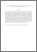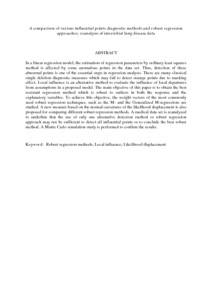# A comparison of various influential points diagnostic methods and robust regression approaches: reanalysis of interstitial lung disease data

## Citation

Bagheri, Arezoo and Midi, Habshah and Ganjali, Mojtaba and Eftekhari, Samaneh (2010) A comparison of various influential points diagnostic methods and robust regression approaches: reanalysis of interstitial lung disease data. Applied Mathematical Sciences, 4 (25-28). pp. 1367-1386. ISSN 1312-885X; ESSN: 1314-7552

## Abstract

In a linear regression model, the estimation of regression parameters by ordinary least squares method is affected by some anomalous points in the data set. Thus, detection of these abnormal points is one of the essential steps in regression analysis. There are many classical single deletion diagnostic measures which may fail to detect strange points due to masking effect. Local influence is an alternative method to evaluate the influence of local departures from assumptions in a proposed model. The main objective of this paper is to obtain the best resistant regression method which is robust to outliers in both the response and the explanatory variables. To achieve this objective, the weight vectors of the most commonly used robust regression techniques, such as the M- and the Generalized M-regressions are studied. A new measure based on the normal curvatures of the likelihood displacement is also proposed for comparing different robust regression methods. A medical data set is reanalyzed to underline that the use of only one alternative detection method or robust regression approach may not be sufficient to detect all influential points or to conclude the best robust method. A Monte Carlo simulation study is performed to confirm the results.Preview
PDF (Abstract)
A comparison of various influential points diagnostic methods and robust regression approaches.pdfView Item